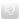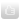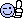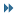# Thread: Visualizing an ADMIXTURE run as a polygonal diagram

1. 12##Visualizing an ADMIXTURE run as a polygonal diagram

When you have a numeric matrix with three columns where the values of the columns add up to a constant on each row, and where there are no negative values, it is possible to visualize the matrix as a ternary plot, where the points within the matrix are drawn inside an equilateral triangle: https://en.wikipedia.org/wiki/Ternary_plot. Basically you can draw an equilateral triangle centered in the origin, with a vector pointing from the origin to each corner of the triangle, and you can then calculate the coordinates of the points as a linear combination of the vectors. Because the rows of the matrix add up to a constant, there is a one-to-one correspondence between coordinates within the triangle and the values in the matrix, since the value of the third column of the matrix is always equal to the values of the first and second column added together and subtracted from the constant.

It is possible to extend the concept of a ternary plot in order to draw a square plot for a matrix with four columns, to draw a pentagon-shaped plot for a matrix with five columns, and so on. However then there is no longer a one-to-one correspondence between points within the polygon and coordinates in the matrix, because for example within a square plot, a point in the middle of the plot can either have 25% of all four components or 50% of two opposite components.

I now selected almost all modern European samples from the 1240K+HO dataset, except I excluded duplicate samples, I excluded one sample from each pair of samples with PI_HAT of .3 or above, and I only included at most 16 samples per population. I then ran ADMIXTURE at each K value from 3 to 8, and I visualized the results as polygonal diagrams.

In the images below, I reordered the admixture components so that I always placed the Kalmyk component at the top of the diagram, because there were no Nenets samples in the dataset I used, so I considered Kalmyks to be the racially purest Europeans. I placed Northern Europeans on the right side of Kalmyks, because there is a cline from Northern Europeans to Kalmyks in Northeastern Europe, and I placed North Caucasians on the left side of Kalmyks, because Nogais are intermediate between Caucasians and Kalmyks.The image below shows population averages from the same ADMIXTURE runs visualized as heatmaps. The clustering is based on a matrix where the columns of each run have been joined into a single wide matrix.

At K=3, the middle component seems like a WHG-like component, because its proportion is the highest in Basques and Lithuanians. The left component is maximal in Kalmyks, but it is also influenced by VURians and Nogais, so even Udmurts have 42% of the left component. Nogais are the only population that has a large proportion of both the first and third components. Nogais from Stavropol are from north of North Caucasus, and Nogais from Astrakhan are from between Kalmykia and Kazakhstan. Compared to them, Nogais from Karachay-Cherkessia (North Caucasus) are closer to Caucasians and less Mongoloid.

At K=4, the middle component breaks off into a Northern European component which is maximal in Estonians and Lithuanians and to a wog component which is maximal in Sardinians. However even Greeks still have 35% of the Caucasian component. Now the proportion of the Mongoloid component also decreases from 42% to 34% in Udmurts.

At K=5, the northern European component splits off into a mysterious ghost component whose proportion is the highest in Arkhangelsk Russians, Gagauzes, and Moldovans. At K=5, Kazan Tatars still have 14% of the Caucasian component but Chuvashes only have 2%. Bashkirs have 8% of the Caucasian component, 36% of the Mongoloid component, and 56% of the Northern European component. However the Bashkir samples are from Jeong et al. 2019 which included both northern and southern Bashkirs, and the southern Bashkir samples had much higher Mongoloid ancestry.

At K=6, the wog component splits off into a Sardinian component and a Maltese component. The Maltese component is more rare, and it has a high percentage only in Maltese, Ashkenazis, and Sicilians. Caucasians were overrepresented in this run, so the Caucasian component also splits into two different components at K=6.

At K=8, a Uralic component that is maximal in Vepsians appears. If these runs would have included more samples of non-Finnic Finno-Permic populations like Saami, Maris, or Komis, the Uralic component might have become more Mongoloid, or it would have appeared at an earlier K value.Download required data and software:

1240K+HO dataset: https://reich.hms.harvard.edu/allen-...cient-dna-data
ADMIXTURE: https://github.com/NovembreLab
Binaries for PLINK 1.9: https://www.cog-genomics.org/plink2/
Compile EIGENSOFT from source: https://reich.hms.harvard.edu/software
Mac binaries for EIGENSOFT 7.2.1: https://drive.google.com/file/d/1H8k...ew?usp=sharing
Mac binaries for an old fork of EIGENSOFT: https://github.com/chrchang/eigensoft

Download the 1240K+HO dataset and run ADMIXURE:

Code:
```curl -LsO reichdata.hms.harvard.edu/pub/datasets/amh_repo/curated_releases/V44/V44.3/SHARE/public.dir/v44.3_HO_public.tar;tar -xf v44.3_HO_public.tar
f=v44.3_HO_public;convertf -p <(printf %s\\n genotypename:\ \$f.geno snpname:\ \$f.snp indivname:\ \$f.ind outputformat:\ PACKEDPED genotypeoutname:\ \$f.bed snpoutname:\ \$f.bim indivoutname:\ \$f.fam)
x=euro5
printf %s\\n Albanian Basque Basque.SDG Belarusian Bulgarian Cretan.DG Croatian Czech English French French.SDG Greek Icelandic Italian_North Italian_South Lithuanian Maltese Moldavian Norwegian Norwegian.DG Orcadian Orcadian.SDG Polish.DG Romanian Russian Russian.SDG Russian_Archangelsk_Krasnoborsky Russian_Archangelsk_Leshukonsky Russian_Archangelsk_Pinezhsky Sardinian Scottish Sicilian Spanish Spanish_North Ukrainian Ukrainian_North Besermyan Estonian Finnish Finnish.DG Hungarian Karelian Mordovian Saami.DG Udmurt Veps Chuvash Gagauz Tatar_Kazan Tatar_Mishar Abazin Adygei Adygei.SDG Avar Balkar Chechen Circassian Darginian Ingushian Kabardinian Kaitag Karachai Kumyk Lak Lezgin Lezgin.DG Ossetian Tabasaran Bashkir Jew_Ashkenazi Kalmyk Nogai_Astrakhan Nogai_Karachay_Cherkessia Nogai_Stavropol>\$x.pop
sed 1d v44.3_HO_public.anno|sort -t\$'\t' -rnk15|awk -F\\t '!a[\$3]++{print\$2,\$8}'|awk 'NR==FNR{a[\$0];next}\$2 in a' \$x.pop ->\$x.temp.pick
plink --allow-no-sex --bfile v44.3_HO_public --keep <(awk 'NR==FNR{a[\$1];next}\$2 in a' \$x.temp.pick v44.3_HO_public.fam) --make-bed --out \$x.temp
plink --allow-no-sex --bfile \$x.temp --genome --out \$x
awk 'FNR>1&&\$10>=.25{print\$2<\$4?\$2:\$4}' \$x.genome|awk 'NR==FNR{a[\$0];next}!(\$1 in a)' - \$x.temp.pick>\$x.pick
plink --allow-no-sex --bfile v44.3_HO_public --keep <(awk 'NR==FNR{a[\$1];next}\$2 in a' \$x.pick v44.3_HO_public.fam) --make-bed --out \$x
plink --allow-no-sex --bfile \$x --indep-pairwise 50 10 .05 --out \$x
plink --bfile \$x --extract \$x.prune.in --make-bed --out \$x.pruned
tav()(awk '{n[\$1]++;for(i=2;i<=NF;i++){a[\$1][i]+=\$i}}END{for(i in a){o=i;for(j=2;j<=NF;j++)o=o FS sprintf("%f",a[i][j]/n[i]);print o}}' "FS=\${1-\$'\t'}")
for k in {3..8};do admixture -j4 -C .1 \$x.pruned.bed \$k;paste -d' ' <(awk 'NR==FNR{a[\$1]=\$2;next}{print\$2,a[\$2]}' \$x.pick \$x.pruned.fam) \$x.pruned.\$k.Q>\$x.\$k;cut -d' ' -f2- \$x.\$k|tav \ >\$x.\$k.ave;done```
Generate polygonal diagrams:

Code:
```library(tidyverse)
library(ggforce)
library(ggrepel)

for(n in c(3,4,5,6,7,8)){
t=read.table(paste0("euro5.",n))
rownames(t)=paste0(t[,2],":",t[,1])
t=t[,-c(1,2)]

columnorder=list(c(2,1,3),c(4,3,2,1),c(2,5,4,3,1),c(4,1,2,3,6,5),c(1,7,5,3,2,6,4),c(2,3,7,4,1,8,5,6))
t=t[,columnorder[[n-2]]]

corners=sapply(c(sin,cos),function(x)head(x(seq(0,2,length.out=n+1)*pi),-1))
corners=corners*min(2/diff(apply(corners,2,range)))
corners[,2]=corners[,2]-mean(range((corners[,2])))

xy=as.data.frame(as.matrix(t)%*%corners)
grid=as.data.frame(rbind(cbind(corners,rbind(corners[-1,],corners[1,])),cbind(corners,matrix(apply(corners,2,mean),ncol=2,nrow=n,byrow=T))))

pop=sub(":.*","",rownames(xy))
pop=sub("\\.(DG|SDG|SG|WGA)","",pop)
centers=aggregate(xy,by=list(pop),mean)
xy\$pop=pop

set.seed(1488)
color=as.factor(sample(seq(1,length(unique(xy\$pop)))))
cl=rbind(c(60,80),c(25,95),c(30,70),c(70,50),c(60,100),c(20,50),c(15,40))
hues=max(ceiling(length(color)/nrow(cl)),2)
pal1=as.vector(apply(cl,1,function(x)hcl(head(seq(15,375,length=hues+1),-1),x,x)))
pal2=as.vector(apply(cl,1,function(x)hcl(head(seq(15,375,length=hues+1),-1),ifelse(x>=60,.5*x,.1*x),ifelse(x>=60,.2*x,95))))

xy\$V1=xy\$V1+runif(nrow(xy))/1e3
xy\$V2=xy\$V2+runif(nrow(xy))/1e3

lims=apply(corners,2,range)+c(-.08,.08)

ggplot(xy,aes(x=V1,y=V2))+
geom_segment(data=grid,aes(x=V1,y=V2,xend=V3,yend=V4),color="gray85",size=.3)+
geom_voronoi_tile(aes(group=0,fill=color[as.factor(pop)],color=color[as.factor(pop)]),size=.07,max.radius=.055)+
geom_label_repel(data=centers,aes(x=V1,y=V2,label=Group.1,color=color,fill=color),max.overlaps=Inf,point.size=0,size=2.3,alpha=.8,label.r=unit(.1,"lines"),label.padding=unit(.1,"lines"),label.size=.1,box.padding=0,segment.size=.3)+
coord_fixed(xlim=lims[,1],ylim=lims[,2],expand=F)+
scale_fill_manual(values=pal1)+
scale_color_manual(values=pal2)+
theme(
axis.text=element_blank(),
axis.ticks=element_blank(),
axis.title=element_blank(),
legend.position="none",
panel.background=element_rect(fill="white")
)

ggsave(paste0(n,".png"),width=7,height=7)
}```
Use ComplexHeatmap to combine heatmaps for different K values (https://jokergoo.github.io/ComplexHe...eference/book/):

Code:
```library(ComplexHeatmap)
library(circlize)
library(colorspace)
library(vegan)

kvals=c(3,4,5,6,7,8)

# columnorder=lapply(kvals,seq)
columnorder=list(c(2,1,3),c(4,3,2,1),c(2,5,4,3,1),c(4,1,2,3,6,5),c(1,7,5,3,2,6,4),c(2,3,7,4,1,8,5,6))

mats=sapply(1:length(kvals),function(i){
t=100*read.table(paste0("euro5.",kvals[i],".ave"),row.names=1)[,columnorder[[i]]]
rownames(t)=sub("Cherkessia","Cher",sub("Russian_Archangelsk_","Rus_Arch_",rownames(t)))
data.frame(aggregate(t,list(sub("\\.(DG|SDG|SG|WGA)|_1|_2","",row.names(t))),mean),row.names=1)
})

png("a.png",w=6000,h=5000,res=144)

maps=sapply(kvals,function(k){
mat=as.matrix(mats[match(k,kvals)][])
Heatmap(
mat,
show_heatmap_legend=F,
show_column_names=F,
show_row_names=F,
clustering_distance_rows="euclidean",
width=ncol(mat)*unit(30,"pt"),
height=nrow(mat)*unit(30,"pt"),
row_dend_width=unit(200,"pt"),
cluster_columns=F,
cluster_rows=reorder(hclust(dist(do.call(cbind,mats))),-mats[][,2]-2*mats[][,1]),
column_title=paste0("K=",k),column_title_gp=gpar(fontsize=24),
right_annotation=rowAnnotation(text1=anno_text(gt_render(rownames(mat),padding=unit(c(2,2,2,2),"mm")),just="left",location=unit(0,"npc"),gp=gpar(fontsize=17))),
col=colorRamp2(seq(0,100,length.out=7),hex(HSV(c(210,210,130,60,40,20,0),c(0,rep(.5,6)),1))),
cell_fun=function(j,i,x,y,w,h,fill)grid.text(sprintf("%.0f",mat[i,j]),x,y,gp=gpar(fontsize=15))
)
})

draw(Reduce(`+`,maps))
dev.off()
system("mogrify -gravity center -trim -border 16 -bordercolor white a.png")```Reply With Quote

2. 1##Its the new way to see genetic, very interesting!Reply With Quote

3. 3##Here's also ADMIXTURE runs for Turkic samples in 1240K+HO. This time I didn't remove related samples with high PI_HAT, so at K=3, the bottom right corner and bottom left corner both include populations with high PI_HAT, like Tubalars, Todzins, Tofalars, and Dolgans. At K=6, there is also one component for Tofalars and another component for Dolgans and Yakuts.Below is a list of the 16 pairs of samples with the highest PI_HAT value. I should've probably at least removed samples with PI_HAT over .35 or .3, but I wanted to demonstrate how the presence of related samples can affect an ADMIXTURE run.

\$ x=turk
\$ printf %s\\n Altaian Altaian_Chelkan Azeri Balkar Bashkir Chuvash Dolgan Gagauz Karachai Karakalpak Kazakh Kazakh_China Khakass Khakass Khakass_Kachin Kumyk Kyrgyz_China Kyrgyz_Kyrgyzstan Kyrgyz_Kyrgyzstan.DG Kyrgyz_Tajikistan Kyrgyz_Tajikstan Nogai Nogai_Astrakhan Nogai_Karachay_Cherkessia Nogai_Stavropol Salar Shor_Khakassia Shor_Mountain Tatar_Kazan Tatar_Mishar Tatar_Siberian Tatar_Siberian_Zabolotniye Todzin Tofalar Tubalar Turkish Turkish.DG Turkish_Balikesir Turkmen Tuvinian Uyghur Uyghur.DG Uzbek Yakut Yakut.DG Yakut.SDG>\$x.pop
\$ awk -F\$'\t' 'NR==FNR{a[\$0];next}\$8 in a&&(!a[\$3]++){print\$2,\$8}' \$x.pop v44.3_HO_public.anno>\$x.pick
\$ plink --bfile v44.3_HO_public --keep <(awk 'NR==FNR{a[\$1];next}\$2 in a' \$x.pick v44.3_HO_public.fam) --make-bed --out \$x
[...]
\$ plink --bfile \$x --genome --out \$x
[...]
\$ awk '{print\$10,\$2,\$4}' \$x.genome|sort -rn|head -n16|awk 'NR==FNR{a[\$1]=\$3;next}{print\$1,a[\$2]":"\$2,a[\$3]":"\$3}' v44.3_HO_public.ind -
0.6302 Tofalar:Vgut8 Tofalar:Vgut12
0.6191 Shor_Khakassia:KHS-035 Shor_Khakassia:KHS-036
0.6139 Tubalar:Tuba23 Tubalar:Tuba24
0.5926 Khakass_Kachin:Khs-493 Khakass_Kachin:Khs-513
0.5816 Tubalar:ALT-116 Tubalar:Tuba2
0.4788 Tofalar:Vgut11 Tofalar:Vgut13
0.4433 Tofalar:Vgut1 Tofalar:Vgut4
0.4302 Tuvinian:Tuvinians86 Tuvinian:Tuvinians111
0.4224 Tubalar:Tuba10 Tubalar:Tuba11
0.3807 Tofalar:Vgut13 Tofalar:Vgut18
0.3765 Karachai:ABA-035 Karachai:ABA-091
0.3675 Tubalar:Tuba21 Tubalar:Tuba1
0.3619 Azeri:AZR-0864 Azeri:AZR-0868
0.3597 Kazakh:KZH-1611 Kazakh:KZH-1750
0.3341 Tatar_Mishar:TTR-272 Tatar_Mishar:TTR-464
0.3264 Tofalar:Vgut11 Tofalar:Vgut15Reply With Quote

4. 3##I now selected ancient samples that had a mean age BP of 6000 or higher and that had at least 400,000 SNPs. I omitted some early Neolithic and WHG samples so they wouldn't be overrepresented. I also omitted Cameroon_SMA and Morocco_Iberomaurusian.

Here's plots of the population averages of the samples, where many populations only consist of a single sample. I joined the columns of the runs at all K values into a single wide matrix. I used the distance matrix of the wide matrix to connect each point to its three closest neighbors, and also to draw convex hulls around the populations based on hierarchical clustering.

At K=3, MA1 and Tyumen_HG are closer to the top pole than to the WHG pole, but it's probably because the top pole includes so many American samples.At K=4, the top pole splits into an American pole and to an East-North Asian pole. There are two paths which connect the WHG pole to the East-North Asian pole. Swedish HGs are connected to Ukraine_N, which is connected to Latvia_MN_o2, which is connected to EHGs, which is connected to WSHGs. Then you can choose from two paths to the East Asian pole: either go from Ust'-Ishim to Tianyuan to China_SEastAsia_Island_EN, or go from USA_Ancient_Beringian to Russia_Kolyma_M to Russia_Siberia_Lena.At K=5, Sunghir splits off into its own pole. In the previous image at K=4, Sunghir had about 50% of the early Neolithic component, 25% of the WHG component, 15% of the East-North Asian component, and 10% of the American component. At K=5, MA1 is also close to the pole of Sunghir. EHGs are a mixture of WHG, American, and Sunghir.At K=6, Iran_N splits off from Turkey_N. Iran_C and Armenia_C are intermediate between them.At K=7, Siberians and Mongolians split off from East Asians, even though they merge again at K=8.At K=8, EHGs split off from WHGs, and SHGs are approximately halfway between EHGs and WHGs. However even Norway_Mesolithic has 100% of the EHG component, because in an ADMIXTURE run like this that includes a relatively small number of samples, often many samples only have 100% of a single component. WSHG is now between EHGs and Americans, but MA1 is between EHGs and Sunghir. Russia_Steppe_Eneolithic is close to the center of the plot, but it just has 44% of the Iran_N component and 44% of the EHG component.In the images above, Ust'-Ishim is close to the center of the plot at most K values. It actually has a balanced mix of different admixture components:Code:
```library(tidyverse)
library(ggforce)
library(ggrepel)

for(k in 3:8){
t=read.table(paste0("hqhg19.",k,"a"),row.names=1)
rownames(t)%<>%sub("\\.(DG|SDG|SG|WGA)","",.)

t=t[,list(c(1,2,3),c(2,3,4,1),c(2,5,1,4,3),c(1,5,4,3,6,2),c(4,5,7,3,1,2,6),c(5,3,1,8,6,4,7,2))[[k-2]]]

corners=sapply(c(sin,cos),function(x)head(x(seq(0,2,length.out=k+1)*pi),-1))
corners=corners*min(2/diff(apply(corners,2,range)))
corners[,2]=corners[,2]-mean(range(corners[,2]))

xy=as.data.frame(as.matrix(t)%*%corners)
grid=as.data.frame(rbind(cbind(corners,rbind(corners[-1,],corners[1,])),cbind(corners,matrix(apply(corners,2,mean),ncol=2,nrow=k,byrow=T))))

joined=sapply(2:8,function(i)read.table(paste0("hqhg19.",i,"a"))[,-1])%>%do.call(cbind,.)%>%set_rownames(rownames(t))
dist=as.data.frame(as.matrix(dist(joined)))
seg=lapply(1:4,function(i)apply(dist,1,function(x)unlist(xy[names(sort(x)[i]),],use.names=F))%>%t%>%cbind(xy))%>%do.call(rbind,.)%>%setNames(paste0("V",1:4))
xy\$k=as.factor(cutree(hclust(dist(joined)),16))

set.seed(1488)
color=as.factor(sample(seq(length(unique(xy\$k)))))
cl=rbind(c(50,90),c(100,80))
hues=max(ceiling(length(color)/nrow(cl)),8)
pal1=as.vector(apply(cl,1,function(x)hcl(head(seq(15,375,length=hues+1),-1),x,x)))

xy\$V1=xy\$V1+runif(nrow(xy))/1e3
xy\$V2=xy\$V2+runif(nrow(xy))/1e3

expand=c(.08,.02)

ggplot(xy,aes(x=V1,y=V2))+
geom_polygon(data=as.data.frame(corners),fill="gray40")+
geom_segment(data=grid,aes(x=V1,y=V2,xend=V3,yend=V4),color="gray50",size=.5)+
geom_mark_hull(aes(group=k,color=k,fill=k),concavity=1000,radius=unit(.3,"cm"),expand=unit(.3,"cm"),alpha=.2,size=.1)+
geom_segment(data=seg,aes(x=V1,y=V2,xend=V3,yend=V4),color="gray20",size=.3)+
geom_point(aes(color=k),size=.5)+
geom_text_repel(aes(label=rownames(xy),color=k),max.overlaps=Inf,force=3,force_pull=2,size=2.3,segment.size=.15,min.segment.length=.15)+
coord_fixed(xlim=(1+expand)*c(-1,1),ylim=(1+expand)*c(-1,1))+
scale_fill_manual(values=pal1)+
scale_color_manual(values=pal1)+
theme(
axis.text=element_blank(),
axis.ticks=element_blank(),
axis.title=element_blank(),
legend.position="none",
panel.background=element_rect(fill="gray30"),
panel.grid=element_blank(),
plot.background=element_rect(fill="gray30",color=NA),
plot.margin=margin(0,0,0,0)
)

ggsave(paste0(n,".png"),width=7,height=7/(Reduce(`/`,1+expand)))
}```Reply With Quote

5. 1##Here's population averages from the European ADMIXTURE run, where each population is linked to its three closest neighbors.

I'm happy that Finns are connected to Kalmyks by only four links: first from Finnish to Russian_Archangelsk_Pinezhsky, then to Besermyan, then to Bashkir, and then to Kalmyk.Reply With Quote

6. 2##Here's runs that include all samples with the suffix ".DG", except for Neanderthals, Denisovans, and samples with the prefix "Ignore_". This time I didn't manually reorder the admixture components at each corner of the polygons, so the order of the components is completely different at different K values.

For example Kusunda and Khonda_Dora are members of the same cluster. At K=10, Kusunda gets its own admixture component which located in the opposite corner from Khonda_Dora, but it doesn't mean that they would actually have a high genetic distance, because the corners of the polygon are in arbitrary order.Reply With Quote

7. 1##I don't know what all this gobbledegook is about but the Kalmyks are no Europeans by any stretch. They are simply 17th century immigrants from Mongolia. By this logic the French Canadians are pure Native Canadians.Reply With Quote

8. 2##It also works with the spreadsheets of calculators. Here's Eurogenes K15 updated:Code:
```library(tidyverse)
library(ggrepel)

t=read.csv("https://pastebin.com/raw/Q3inavNV",row.names=1,check.names=F)
t=t/100
n=ncol(t)

corners=sapply(c(sin,cos),function(x)head(x(seq(0,2,length.out=n+1)*pi),-1))
corners=corners*min(2/diff(apply(corners,2,range)))
corners[,2]=corners[,2]-mean(range(corners[,2]))

xy=as.data.frame(as.matrix(t)%*%corners)
grid=as.data.frame(rbind(cbind(corners,rbind(corners[-1,],corners[1,])),cbind(corners,matrix(apply(corners,2,mean),ncol=2,nrow=n,byrow=T))))

dist=as.data.frame(as.matrix(dist(t)))
seg=lapply(1:4,function(i)apply(dist,1,function(x)unlist(xy[names(sort(x)[i]),],use.names=F))%>%t%>%cbind(xy))%>%do.call(rbind,.)%>%setNames(paste0("V",1:4))
xy\$k=as.factor(cutree(hclust(dist(t)),16))

hue=c(0,30,60,90,130,180,210,240,280,320)
pal1=c(hex(HSV(hue[-c(8,9)],.5,1)),hex(HSV(hue,.25,1)))

expand=c(.02,.02)

angle=head(seq(360,0,length.out=n+1),-1)
angle=ifelse(angle>90&angle<=270,angle+180,angle)

ggplot(xy,aes(x=V1,y=V2))+
geom_polygon(data=as.data.frame(corners),fill="gray40")+
geom_text(data=as.data.frame(corners),aes(x=1.04*V1,y=1.04*V2),label=names(t),size=3.2,angle=angle,color="gray80")+
geom_segment(data=grid,aes(x=V1,y=V2,xend=V3,yend=V4),color="gray50",size=.4)+
geom_mark_hull(aes(group=k,color=k,fill=k),concavity=1000,radius=unit(.3,"cm"),expand=unit(.3,"cm"),alpha=.15,size=.15)+
geom_segment(data=seg,aes(x=V1,y=V2,xend=V3,yend=V4),color="gray20",size=.3)+
geom_point(aes(color=k),size=.5)+
geom_text(aes(label=rownames(xy),color=k),size=2.2,vjust=-.6)+
# geom_text_repel(aes(label=rownames(xy),color=k),max.overlaps=Inf,force=4,force_pull=2,size=2.2,segment.size=.2,min.segment.length=.2,box.padding=.05)+
coord_fixed(xlim=(1+expand)*c(-1,1),ylim=(1+expand)*c(-1,1))+
scale_fill_manual(values=pal1)+
scale_color_manual(values=pal1)+
theme(
axis.text=element_blank(),
axis.ticks=element_blank(),
axis.title=element_blank(),
legend.position="none",
panel.background=element_rect(fill="gray30"),
panel.grid=element_blank(),
plot.background=element_rect(fill="gray30",color=NA,size=0),
plot.margin=margin(0,0,0,0)
)

ggsave("t/a.png",width=9,height=9/(Reduce(`/`,1+expand)))```Originally Posted by LetoI don't know what all this gobbledegook is about but the Kalmyks are no Europeans by any stretch. They are simply 17th century immigrants from Mongolia. By this logic the French Canadians are pure Native Canadians.
Europe is a multiracial continent that is populated by the wog race, the white race, and the Turco-Uralo-Mongolic race.

Parts of Europe have been populated by peoples with 50% or higher Mongoloid ancestry since at least the time of Bolshoy Oleni Ostrov almost 4,000 years ago. And even before Nenetses, the area of Nenetsia was inhabited by Sikhirtya, who were described as having Mongoloid appearance (https://avaldsnes.info/en/informasjon/hjor/).

Even before the Kalmyk expansion, the area of Kalmykia was part of the Xacitarxan Khanate.

Some Kalmyks like this pass as Europeans (Kalmyk or Nenets or Kazakh) but not as unmixed East Asians:

Spoiler!Reply With Quote

9. 2##Here's Dodecad K12b:The clustering would work better if I was somehow able to take the table of FST distances between each component into account:Reply With Quote

10. 1##Originally Posted by KomintasavaltaThe clustering would work better if I was somehow able to take the table of FST distances between each component into account:I tried something like this recently:

Code:
```a <- read.table("results.csv", header = TRUE, row.names=1)
b <- read.table("fst_distances.csv", header = TRUE, row.names=1)

a <- as.matrix(a)
b <- as.matrix(b)

c <- a %*% b

write.table(c, file = "fst_scaled.txt")```
i didn't like the result. Basically all Europeans end up more similar to each other, and some Hungarians with a tiny bit of Asian were bigger outliers.Reply With Quote

Page 1 of 4 1234 Last#### Thread Information

##### Users Browsing this Thread

There are currently 1 users browsing this thread. (0 members and 1 guests)

####Posting Permissions

• You may not post new threads
• You may not post replies
• You may not post attachments
• You may not edit your posts
•